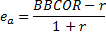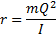Sports Science Laboratory Bat-Ball Science Normalizing Bat Performance

## Normalizing Bat Performance

Normalizing relations are used to minimize error in measuring bat performance. The experimental error can be due to variation in ball properties and test speeds. While they complicate the formulas used to calculate performance, they improve the experimental repeatability.

It is desirable to make the laboratory measures of performance as accurate and repeatable as possible. Small variations in ball weight, stiffness, and elasticity can have a measurable effect on bat performance.

Balls with higher stiffness or COR will increase the BBCOR. This influence is limited by prescribing the type of ball used in the test. Variation within a ball model can still affect the result, however. The BPF assumes a linear dependence of the ball COR on the BBCOR. Recent experiments and models indicate that the dependence of the ballCOR on the BBCOR is non-linear, however. Results show that the BPF over-corrects for the ball COR; in many cases by the original amount of the ball COR on theBBCOR.

A new model has been developed to account for variation in ball COR and stiffness. [n] The BBCOR is normalized to a ball having a standard CCOR (CCORs) and a standard stiffness (ks) according to

where BBCORn is the normalized BBCOR and rN is given by

Figure 11.1 compares experimental test results without normalizing (blue) with values that were normalized using Eq. (10.2) (black) and values that were normalized using Eq. (11.1) (red). As expected, the unnormalized results increase with ball stiffness (Fig. a) and ball COR (Fig. b). Ideally the normalized results would fall on a horizontal line with no dependence on ball stiffness or CCOR. Equation (11.1) is observed to significantly reduce the effect of ball stiffness and almost eliminate the effect of ball CCOR, while Eq. (10.2) provides no noticeable improvement.

Figure 11.1.  Results for normalizing the BBCOR (e). Unnormalized values are in blue. Normalized values using the BPF are in black. Normalized values using Eq. (11.1) are in red. The dotted lines are linear fits to the data.  Figure (a) considers balls of different stiffness, while figure (b) considers balls of different COR.

The collision efficiency, and measures that use it, are affected by variation in the ball weight. The collision efficiency and BBCOR are related according to [o](11.3)

where, for a pivoted bat,(11.4)

Thus, the collision efficiency may be normalized to a nominal ball weight from Eq. (11.3) if a nominal ball weight (m) is used in Eq. (11.4). While this is a more complicated path than Eq. (10.3), it reduces the effect of small variations in the weight of test balls on bat performance. An example of weight normalizing is presented in Fig. 11.2 for four bats tested with balls of three weights. Without normalizing, the performance of each bat decreases with increasing ball weight. With normalizing, the performance of each bat is nearly unchanged with test ball weight. (The variation in test ball weight was larger for this illustrative example than occurs in certified bat performance tests.)

Fig. 11.2. Comparison of normalizing on the performance of four bats as a function of test ball weight.

[n] Nathan, A. M. and Smith, L. V., 2009 “Effect of Ball Properties On the Ball-Bat Coefficient of Restitution,” 4th Asia-Pacific Congress on Sports Technology, Honolulu, Hawaii.
[o] Alan M. Nathan, Am. J. Phys. 71, 134 (2003)